Dominic Savio

•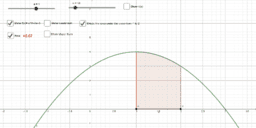Riemann Sum - Area Under a Curve

Activity

Dominic Savio

•Riemann Sum

Activity

Dominic Savio

•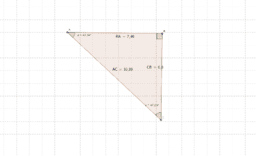Trigonometric Ratios - Investigation

Activity

Dominic Savio

•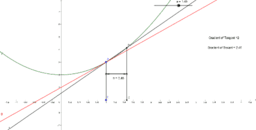Gradient of a curve at a point - Derivative

Activity

Dominic Savio

•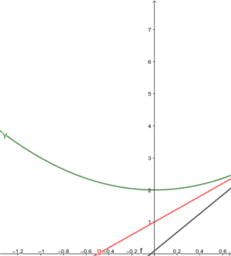derivative from first principle

Activity

Dominic Savio

•Parallel and Perpendicular lines

Activity

Dominic Savio

•Number of roots of quadratic functions of an equation

Activity

Dominic Savio

•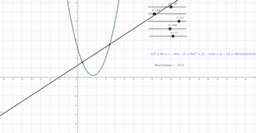Solving system of non linear equations Simultaneously

Activity

Dominic Savio

•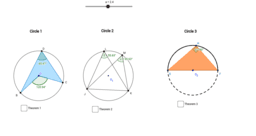Circle Theorems: Angle properties

Activity

Dominic Savio

•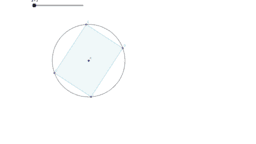Circle Theorems: Angles of Cyclic Quadrilateral

Activity

Dominic Savio

•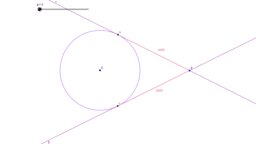Circle Theorems: Tangent Property 2

Activity

Dominic Savio

•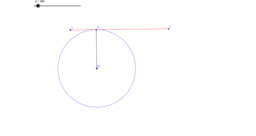Circle Theorems: Tangent property 1

Activity

Dominic Savio

•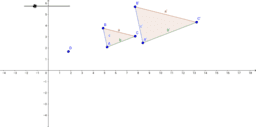Similarity of triangles sides proportionality

Activity

Dominic Savio

•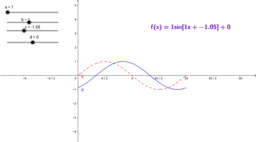Period, Amplitude & Shift

Activity

Dominic Savio

•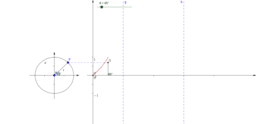Trigonometric Functions Graph - Tan x

Activity

Dominic Savio

•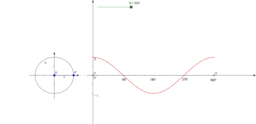Trigonometric Functions Graph- cos x

Activity

Dominic Savio

•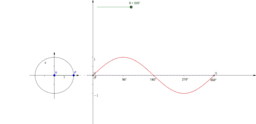Trigonometric Functions Graph - Sinx

Activity

Dominic Savio

•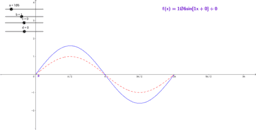Period, Amplitude and Shift

Activity

Dominic Savio

•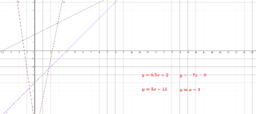Identifying slopes of lines from Equations

Activity

Dominic Savio

•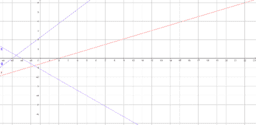Slope of Inclined Lines

Activity

Dominic Savio

•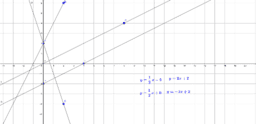Identifying gradient and intercept of lines

Activity

Dominic Savio

•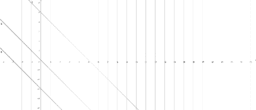Naming lines 2

Activity

Dominic Savio

•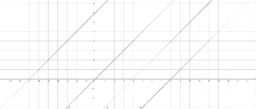Naming the lines

Activity

Dominic Savio